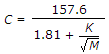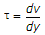# Civil Engineering - Hydraulics

### Exercise :: Hydraulics - Section 6

11.

 A. area divided by the square of wetted perimeter B. area divided by wetted perimeter C. wetted perimeter divided by area D. square root of the area.

Explanation:

No answer description available for this question. Let us discuss.

12.

The discharg formula Q = Cd 2gH x A is used for rectangular

 A. small orifices only B. large orifices only C. small and large orifices only D. for all types of orifices.

Explanation:

No answer description available for this question. Let us discuss.

13.

Chezy's constantis suggested by

 A. Bazin B. Kutter C. Manning D. Powell.

Explanation:

No answer description available for this question. Let us discuss.

14.

The acceleration f required to accelerate a rectangular tank containing water horizontally so that the slope of its free surface is 45°, is

 A. g/2 B. g C. 2 g D. 2.5 g E. 3 g.

Explanation:

No answer description available for this question. Let us discuss.

15.

Pick up the correct statement from the following :

 A. The fluids which followare known as Newtonian fluids B. The fluids which do not follow the linear relationship between shear and rate of strain are known as non Newtonian fluids C. The substances which flow after yield strains, are known as plastics D. all the above.